Mathematics
Easy

Question

# The triangle sum theorem states that the sum of the angles of a triangle equal how many degrees?

## 90°30°150°180°Hint:

## The correct answer is: 180°

Mathematics

Mathematics

Mathematics

### Do we have enough information to say these two triangles are congruent? If so, what allows us to say this?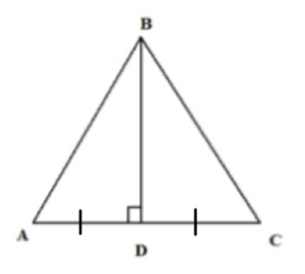Mathematics

Mathematics

Mathematics

### State if the two triangles are congruent. If they are, state how you know.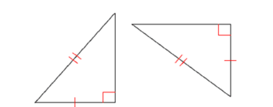Mathematics

Mathematics

### Complete the congruence statement: ∆ BCA =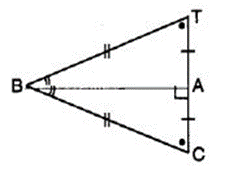Congruent triangles are triangles that have same size and shape. This suggests us that the corresponding sides are equal and corresponding angles are equal.
When two triangles are congruent, all of their corresponding sides and angles are equal.

### Complete the congruence statement: ∆ BCA =Congruent triangles are triangles that have same size and shape. This suggests us that the corresponding sides are equal and corresponding angles are equal.
When two triangles are congruent, all of their corresponding sides and angles are equal.

Mathematics

### We want to show that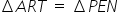. We have to use SAS criterion. We have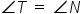, RT = EN, then____________.

SAS congruence is the term which is also known as Side Angle Side congruence. In this, triangles are congruent if any pair of corresponding sides and their included angles are equal in both triangles.

### We want to show that. We have to use SAS criterion. We have, RT = EN, then____________.

SAS congruence is the term which is also known as Side Angle Side congruence. In this, triangles are congruent if any pair of corresponding sides and their included angles are equal in both triangles.Mathematics

Mathematics

Mathematics

### ∆ PQR is congruent to ∆ STU (in figure), then length of TU is ___________.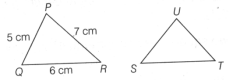Congruent triangles are triangles that have same size and shape. This suggests us that the corresponding sides are equal and corresponding angles are equal.
When two triangles are congruent, all of their corresponding sides and angles are equal.

### ∆ PQR is congruent to ∆ STU (in figure), then length of TU is ___________.Congruent triangles are triangles that have same size and shape. This suggests us that the corresponding sides are equal and corresponding angles are equal.
When two triangles are congruent, all of their corresponding sides and angles are equal.Mathematics

Mathematics

Mathematics

### The symbol of correspondence is

The double-sided arrow symbol is used to denote correspondence

### The symbol of correspondence is

The double-sided arrow symbol is used to denote correspondence#### With Turito Foundation.#### Get an Expert Advice From Turito.